What does it do?

Counts the number of cells that matches your specified condition

Formula breakdown:

=COUNTIF(range, criteria)

What it means:

=COUNTIF(range of cells to check, condition to check against)

Do you have a scenario where you want to count the number of cells that match a specific condition?

I’m sure you do!  There is a simple way to count this with Excel’s COUNTIF formula!

The COUNTIF formula is very flexible indeed, so let us try to count the following from our Excel worksheet:

• Number of cells greater than 2
• Number of cells that have a Yellow value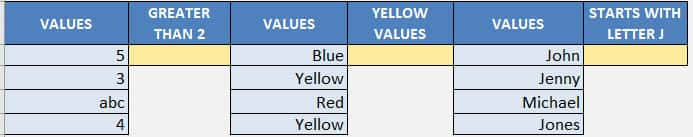I explain how you can do this below:STEP 1: We need to enter the COUNTIF function in a blank cell:

## =COUNTIF(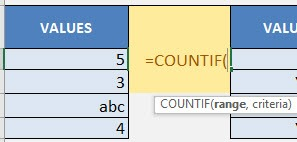STEP 2: The COUNTIF arguments:

## range

What is the range of values that you want to check against your condition?

## =COUNTIF(A9:A12,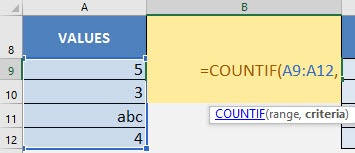## criteria

What is the condition that you want to check against?

For our 1st example, we want to count the number of values greater than 2.

## =COUNTIF(A9:A12, “>2”)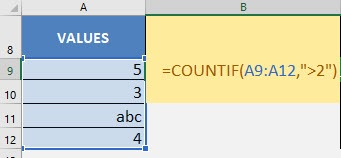You now have your count of numbers greater than 2!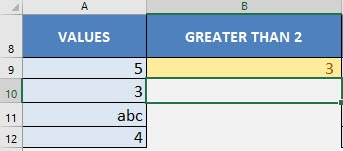STEP 3: Now let us try for counting the number of Yellow values:

## =COUNTIF(C9:C12, “Yellow”)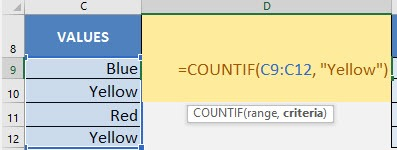You now have your count of values that have the Yellow text!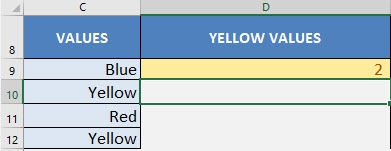STEP 4: Now let us try for counting the number of names starting with the Letter J:

Let us use the wildcard expression J*

* signifies a wildcard character i.e. Return any value that begins with a J

## =COUNTIF(E9:E12, “J*”)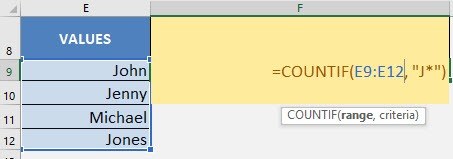You now have your count of values that have a starting letter of J!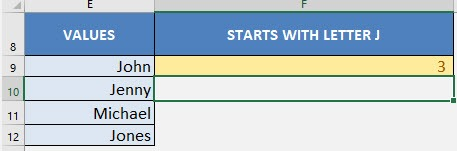CountIf Formula in Excel